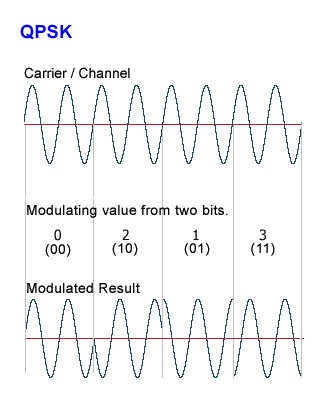# bandwidth

(redirected from Banwith)
Also found in: Dictionary, Thesaurus, Medical, Financial.

## bandwidth

1. The range of frequencies over which an instrument, such as a radio telescope, is sensitive. A wide bandwidth gives good sensitivity to sources that emit over a wide range of frequencies (e.g. by synchrotron emission), whereas a narrow bandwidth gives good sensitivity to spectral lines.
2. (coherence bandwidth) The range of frequencies over which electromagnetic or other emissions from a source maintain coherence.

## bandwidth

[′band‚width]
(communications)
The difference between the frequency limits of a band containing the useful frequency components of a signal.
A measure of the amount of data that can travel a communications path in a given time, usually expressed as thousands of bits per second (kbps) or millions of bits per second (Mbps).

## bandwidth

(communications)
The difference between the highest and lowest frequencies of a transmission channel (the width of its allocated band of frequencies).

The term is often used erroneously to mean data rate or capacity - the amount of data that is, or can be, sent through a given communications circuit per second.

## bandwidth

(1) Computer people may use the term for capability and time. For example, "not enough bandwidth to get the job done" means not enough staff or time to do it. Its true meaning follows.

(2) The transmission capacity of an electronic pathway such as a communications line, computer bus or computer channel. Digital bandwidth is the number of pulses per second measured in bits per second (bps). For example, Ethernet transmits at different speeds, including 10 Mbps, 100 Mbps and 1000 Mbps (see Mbps and baseband).

When transmitting alternating frequencies, as with all wired analog, many wired digital and most wireless communications, the bandwidth is the difference between the highest and lowest frequencies, measured in cycles per second, or Hertz (Hz). For example, 802.11n Wi-Fi transmits in 20 MHz and 40 MHz channels within the 2.4 GHz and 5 GHz bands. The 20 and 40 MHz channel frequencies are the bandwidths, and each channel is divided into subchannels.

From Hertz to Bits - A Complicated Process
When using alternating frequencies for digital transmission, the frequencies are modified (modulated) by the digital input. Using the 802.11n Wi-Fi example, the resulting bit rate can range from 6.5 Mbps to 600 Mbps. This extremely wide range is determined by the signal strength and interference in the environment at any given moment. Any one of more than 30 combinations of channel bandwidth (20, 40 MHz), modulation scheme, error correction rate, channel spacing and number of antennas may be selected, on a packet-by-packet basis. See modulation, video bandwidth, space/time and bandwidth junkie.

Bandwidth in Hertz to Bandwidth in BitsThis quadrature PSK (QPSK) example is one of the simplest modulation schemes. Each set of two input bits modifies the carrier into four phase angles. The amplitude remains constant, unlike QAM modulation, in which the amplitude is varied (see QAM).Site: Follow: Share:
Open / Close